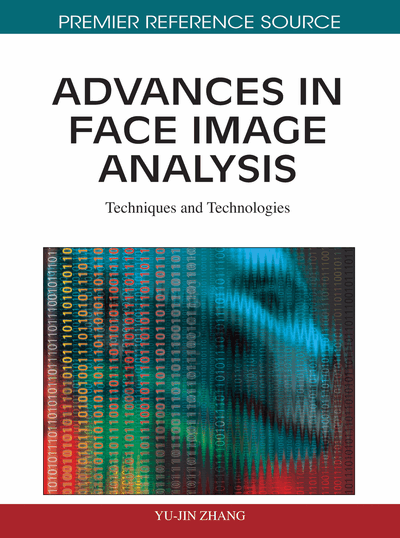# Feature Selection in High Dimension

Sébastien Gadat (Université Paul Sabatier, France) and Sébastien Gadat (Université Paul Sabatier, France)
DOI: 10.4018/978-1-61520-991-0.ch006
Available
\$37.50
No Current Special Offers

## Abstract

Variable selection for classification is a crucial paradigm in image analysis. Indeed, images are generally described by a large amount of features (pixels, edges …) although it is difficult to obtain a sufficiently large number of samples to draw reliable inference for classifications using the whole number of features. The authors describe in this chapter some simple and effective features selection methods based on filter strategy. They also provide some more sophisticated methods based on margin criterion or stochastic approximation techniques that achieve great performances of classification with a very small proportion of variables. Most of these “wrapper” methods are dedicated to a special case of classifier, except the Optimal features Weighting algorithm (denoted OFW in the sequel) which is a meta-algorithm and works with any classifier. A large part of this chapter will be dedicated to the description of the description of OFW and hybrid OFW algorithms. The authors illustrate also several other methods on practical examples of face detection problems.
Chapter Preview
Top

## Introduction

High dimensional data Most of nowadays encountered examples of face analysis tasks involve high-dimensional input variables. To detect faces in an image, we usually consider the set of all possible gray-level pixels of the image as informative features. In this case, features are considered as “low level” features contrary to more sophisticated “high level features” built from linear or non linear combination of the gray-level pixels such as linear filters, edges (mostly coming from some thresholding step), Fourier and Wavelet coefficients, principal components …

In the case of high level features, the number of variables may thus become very large and can even exceed the number of images. Imagine for instance one thousand samples of images described by 60 × 85 pixels, signals are thus defined by 5100 low-level features and the number of samples become rapidly too small to describe efficiently the data. Consequently, algorithms involving face detection tasks must generally solve some large dimensional problems while a large amount of variables may not be so good to reach accuracy and robustness properties as we will see in the next paragraph.

In this chapter, we focus on the problem of selecting features associated to the detection of “faces” (versus “non-faces”) when images are described by high-dimensional signals.

A statistical remark and the curse of dimensionality Let us first discuss in further details why abundance of variables can significantly harm classification in the context of face detection. From a statistical point of view, it is important to remove some irrelevant variables which act as artificial noise in data, especially in the case of images, and limit accuracy of detection tasks.

Moreover, in high dimensional spaces, we face the curse of dimensionality and it is generally impossible to draw some reliable conclusions from databases built with little number of examples regarding some large number of features. This phenomenon has been first pointed by Bellman (1961). Let us describe briefly this statistical property which can be summarized as the exponential growth of hyper-volume as a function of dimensionality. Consider for instance the learning task of face detection. The signal is denoted I while the presence of a face in the signal is Y (Y = 1 when a face is present and Y = 0 otherwise). A good statistical prediction of Y given I corresponds to a good knowledge of the joint law Y|X. If we called the dimension of the space Ed where I lives, the problem is equivalent to find a correct interpolation of this joint law given a Learning Set of size N when the samples are drawn in the space Ed. As d increases, the number of samples N necessary to build a design of fixed precision increases exponentially. For instance, N = 100 uniformly-spaced sample points suffice to sample a unit interval of dimension one with no more than 0.01 distance between points although an equivalent sampling of a 10-dimensional (d = 10) unit hypercube with a lattice spacing of 0.01 between adjacent points would require N = 1020 sample points. Thus, it will be exponentially hardest to approximate the joint law Y|X when d is increasing.

In addition, let us remind the classical Bias/Variance trade-off (see (Geman, Bienenstock, and Doursat, 1992). If one want to predict Y with a function f applied to signal I using observations in a Learning Set D, the square loss decomposition for the prediction f(X) is given as

(1)

In the former decomposition, the bias is typically a non increasing function of the dimension d while the variance term may drastically increase with d. It is thus necessary to follow a Bias/Variance trade-off to find a good prediction f and a good complexity d. One of the goals of the selection of features is to reduce this phenomenon by restricting the set of features to the “good” ones.

At last, we can notice some efficiency issues, since the speed of many classification algorithms is largely improved when the complexity of the data is reduced. For instance, the complexity of the q-nearest neighbour algorithm varies proportionally with the number of variables. In some cases, the application of classification algorithms like Support Vector Machines described in Vapnik (1998) or q-nearest neighbours on the full features space is not possible or realistic due to the time needed to apply the decision rule.

## Complete Chapter List

Search this Book:
Reset